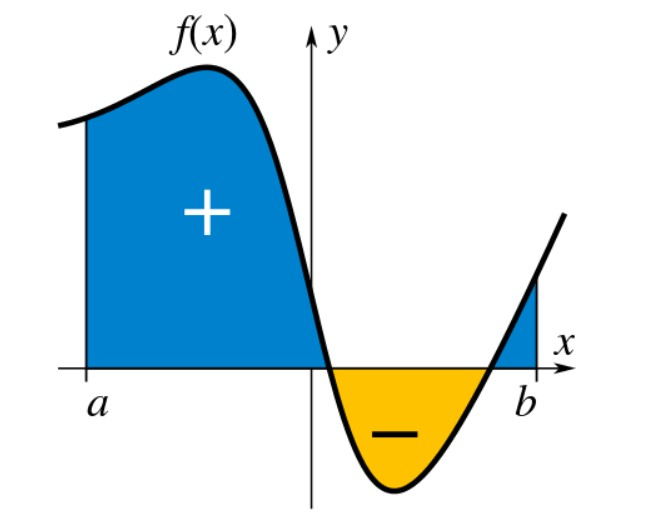# Antiderivative Calculator

Enter the function, variable, upper, and lower bound limit. Select definite or indefinite integral option. Hit the Calculate button to find the antiderivative using antiderivative calculator.

+
x
/
(
)

Give Us Feedback

## Antiderivative calculator with steps

Online integral (antiderivative) calculator is a tool that evaluates the integral of a given function with respect to a variable. It also calculates the definite as well as indefinite integral for the given function.

This integral calculator also shows the steps of integration for every calculation.

## What is an integral?

An integral can be defined as,

An integral assigns numbers to functions in a way that can define volume, area displacement and even probability. Integral is inverse function of derivative, which is why, it is generally referred to as antiderivative.

The process of finding integral is known as integration. It is used to find the area under the curve. The symbol of integration or antiderivative is ∫.## How to evaluate an integral?

Example: Evaluate the following integral.

∫ (6x + 2) dx

Solution:

Step 1: Apply linearity to the function.

= 6 ∫ x dx + 2 ∫ 1 dx ------- 1

Step 2: Solve 6 ∫ x dx and 2 1 dx separately and place the values in above equation (1).

6 ∫ x dx

Apply power rule.6 ∫ x dx = 6x2/2 = 3x2

2 1 dx

Apply constant rule ∫a dx = ax + C.

2 ∫ 1 dx = 2x + C

Step 3: Put the solved integrals in equation (1).

= 6 ∫ x dx + 2 ∫ 1 dx

= 3x2 + 2x + C

The integral solver above performs all of these steps and shows you the complete calculation for your ease.

### Other Languages